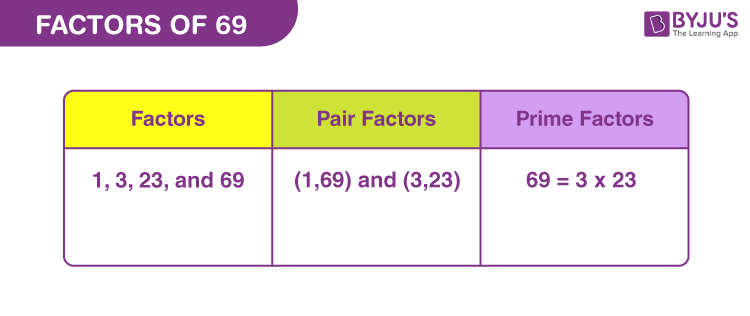# Factors of 69

In Maths, factors of 69 are the natural numbers that divide 69 into equal parts. 69 is a factor of itself. A factor will divide the original number evenly, without leaving any remainder. 69 is an odd composite number, thus has more than two factors.

Let us find the factors of 69 along with pair factors and prime factors, using simple division and prime factorisation methods, respectively. Also, get solved examples here.## How to Find the Factors of 69?

The factors of 69 can be evaluated by dividing the original by the natural numbers. If the remainder is zero, after division, then the divisor becomes the factor.

69 ÷ 1 = 69 [Remainder = 0]

69 ÷ 3 = 23 [Remainder = 0]

69 ÷ 23 = 3 [Remainder = 0]

69 ÷ 69 = 1 [Remainder = 0]

Thus, the factors of 69 are 1, 3, 23 and 69.

## Pair Factors of 69

The pair factors are the integers that are multiplied together to get the original number.

1 × 69 = 69

3 × 23 = 69

Therefore, the pair factors are (1, 69) and (3, 23).

Now, if we consider the negative integers as a pair factor of 69, then the product of two negative integers will result in the original number.

-1 × -69 = 69

-3 × -23 = 69

Therefore, the negative pair factors are (-1, -69) and (-3, -23).

## Prime Factorisation of 69

The prime factors of 69 can be determined by the prime factorisation method. In this method, we divide the original number by the prime numbers unless we get a quotient equal to 1.

List of prime numbers (Between 1 and 69) :

 2, 3, 5, 7, 11, 13, 17, 19, 23, 29, 31, 37, 41, 43, 47, 53, 59, 61, 67

Step 1: From the above list, we can see 69 is divisible by the prime number 3.

69 ÷ 3 = 23

Step 2: Since 23 is itself a prime number, therefore, it is divisible by itself.

23 ÷ 23 = 1

Therefore, the prime factors of 69 are 3 and 23.

 Prime factorisation of 69 = 3 x 23 Exponential Form = 31 x 231

## Solved Examples

Q.1: Find the sum of all the factors of 69.

Solution: The factors of 69 are 1, 3, 23 and 69 .

Sum = 1 + 3 + 23 + 69 = 96

Therefore, 96 is the required sum.

Q.2: What are the common factors of 39 and 69?

Answer: Let us first write the factors of both the numbers.

Factors of 69 → 1, 3, 23, 69

Factors of 39 → 1, 3, 13, 39

Therefore, common factors of 39 and 69 are 1 and 3.

Q.3: What is the GCF of 99 and 69?

69 → 1, 2, 43, 69

99 → 1, 3, 9, 11, 33, 99

Therefore, the greatest common factor (GCF) is 1.

### Practice Problems

1. Find the average of factors of 69.
2. Check if 69 and 79 are co-primes.
3. What is the GCF of 81 and 69?
4. Geeta has two sheets of paper, one of 69 cm in length and another of 86. Find the maximum length of the sheet into which she can divide both sheets.

## Frequently Asked Questions on Factors of 69

### What are the factors of 69?

There are a total of four factors of 69. These factors are 1, 3, 23 and 69.

### What is the prime factor form of 69?

The prime factorisation of 69 is: 3 x 23.

### Is 69 a prime number?

69 is not a prime number but a composite number. A prime has only two factors but 69 has four factors.

### What are the multiples of 69?

The first ten multiples of 69 are 69, 138, 207, 276, 345, 414, 483, 552, 621 and 690.

### Is 69 a perfect square?

69 is not a perfect square. The square root of 69 is not a whole number.